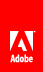# Using the Math class with drawing methods

Flash Player 9 and later, Adobe AIR 1.0 and later

 A Graphics object draws circles and squares, but can also draw more complex forms, particularly when the drawing methods are used in combination with the properties and methods of the Math class. The Math class contains constants of common mathematical interest, such as Math.PI (approximately 3.14159265...), a constant for the ratio of the circumference of a circle to its diameter. It also contains methods for trigonometry functions, including Math.sin() , Math.cos() , and Math.tan() among others. Drawing shapes using these methods and constants create more dynamic visual effects, particularly when used with repetition or recursion. Many methods of the Math class expect circular measurements in units of radians rather than degrees. Converting between these two types of units is a common use of the Math class: ```var degrees = 121; var radians = degrees * Math.PI / 180; trace(radians) // 2.111848394913139``` The following example creates a sine wave and a cosine wave, to highlight the difference between the Math.sin() and Math.cos() methods for a given value. ```var sinWavePosition = 100; var cosWavePosition = 200; var sinWaveColor:uint = 0xFF0000; var cosWaveColor:uint = 0x00FF00; var waveMultiplier:Number = 10; var waveStretcher:Number = 5; var i:uint; for(i = 1; i < stage.stageWidth; i++) { var sinPosY:Number = Math.sin(i / waveStretcher) * waveMultiplier; var cosPosY:Number = Math.cos(i / waveStretcher) * waveMultiplier; graphics.beginFill(sinWaveColor); graphics.drawRect(i, sinWavePosition + sinPosY, 2, 2); graphics.beginFill(cosWaveColor); graphics.drawRect(i, cosWavePosition + cosPosY, 2, 2); }```Twitter™ and Facebook posts are not covered under the terms of Creative Commons.// Ethnio survey code removed